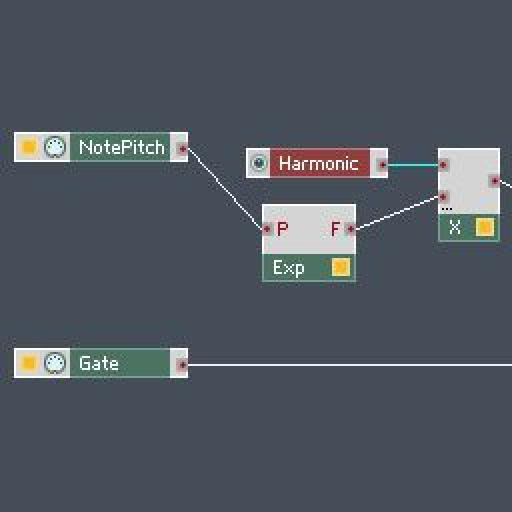# Creating Partials in Native Instruments' Reaktor

David Earl shows how to use Reaktor to convert logarithmic pitch to frequency, create a harmonic series and control it all using one knob! A little essential theory followed by all the practical steps

In Additive Synthesis, sine waves are added together to make more complex sounds. The Fourier Theory states that any complex wave can be broken down into sinusoidal components, so Additive Synthesis is the way to build complex sounds from the ground up. A sine wave has no harmonics. Each sine wave we add in to the mix is called a partial or overtone.

Common waves used in synthesis, like the sawtooth wave, are built off partials that follow a pattern called the harmonic series. The harmonic series is built from the fundamental pitch frequency. The partials are calculated by multiplying the fundamental by 1,2,3,4,5...etc. The amplitude of the upper partials is less than the fundamental pitch.

Example:

• Fundamental is 100hz
• 2nd Harmonic = 200hz
• 3rd Harmonic = 300hz
• 4th Harmonic = 400hz

• A sawtooth wave is built from all of the partials of the harmonic series
• A square wave is all odd harmonics
• A triangle wave is all odd harmonics as well, but with the upper partials attenuated in amplitude quite a bit.

In Reaktor, we are going to convert logarithmic pitch to frequency. We will then use multiplication to get our harmonic series quickly and easily using a control knob.

### Step 1

Open a new ensemble in Reaktor and open Structure view of the Default instrument. Control-Click in the workspace and select '

## Discussion

#### Want to join the discussion?

Create an account or login to get started!